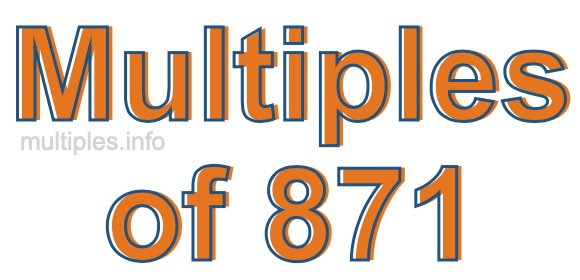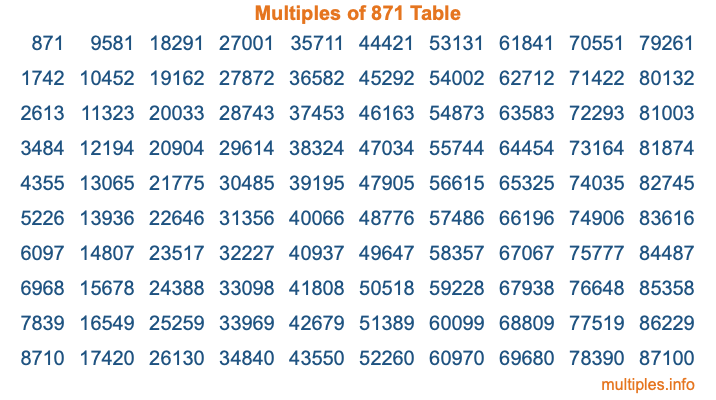Multiples of 871Welcome to the Multiples of 871 page. Here we will first teach you everything you will ever need to know about the multiples of 871, and then give you a study guide summary of everything we taught you to make sure you remember it all. Use this page to look up facts and learn information about the multiples of 871. This page will make you a multiples of eight hundred seventy-one expert!

Definition of Multiples of 871
Multiples of 871 are all the numbers that when divided by 871 equal an integer. Each of the multiples of 871 are called a multiple. A multiple of 871 is created by multiplying 871 by an integer.

Therefore, to create a list of multiples of 871, you start with 1 multiplied by 871, then 2 multiplied by 871, then 3 multiplied by 871, and so on for as long as you want. Thus, the list of the first five multiples of 871 is 871, 1742, 2613, 3484, and 4355. To see a larger list of multiples of 871, see the printable image of Multiples of 871 further down on this page. We also have a category where you can choose any nth multiple of 871.

Multiples of 871 Checker
The Multiples of 871 Checker below checks to see if any number of your choice is a multiple of 871. In other words, it checks to see if there is any number (integer) that when multiplied by 871 will equal your number. To do that, we divide your number by 871. If the the quotient is an integer, then your number is a multiple of 871.

Is  a multiple of 871?

Least Common Multiple of 871 and ...
A Least Common Multiple (LCM) is the lowest multiple that two or more numbers have in common. This is also called the smallest common multiple or lowest common multiple and is useful to know when you are adding our subtracting fractions. Enter one or more numbers below (871 is already entered) to find the LCM.

Check out our LCM Calculator if you need more details about the Least Common Multiple or if you need the LCM for different numbers for adding and subtraction fractions.

nth Multiple of 871
As we stated above, 871 is the first multiple of 871, 1742 is the second multiple of 871, 2613 is the third multiple of 871, and so on. Enter a number below to find the nth multiple of 871.

th multiple of 871

Multiples of 871 vs Factors of 871
871 is a multiple of 871 and a factor of 871, but that is where the similarities end. All postive multiples of 871 are 871 or greater than 871. All positive factors of 871 are 871 or less than 871.

Below is the beginning list of multiples of 871 and the factors of 871 so you can compare:

Multiples of 871: 871, 1742, 2613, 3484, 4355, etc.

Factors of 871: 1, 13, 67, 871

As you can see, the multiples of 871 are all the numbers that you can divide by 871 to get a whole number. The factors of 871, on the other hand, are all the whole numbers that you can multiply by another whole number to get 871.

It's also interesting to note that if a number (x) is a factor of 871, then 871 will also be a multiple of that number (x).

Multiples of 871 vs Divisors of 871
The divisors of 871 are all the integers that 871 can be divided by evenly. Below is a list of the divisors of 871.

Divisors of 871: 1, 13, 67, 871

The interesting thing to note here is that if you take any multiple of 871 and divide it by a divisor of 871, you will see that the quotient is an integer.

Multiples of 871 Table
Below is an image of the first 100 multiples of 871 in a table. The table is in chronological order, column by column. The first column has the first ten multiples of 871, the second column has the next ten multiples of 871, and so on.The Multiples of 871 Table is also referred to as the 871 Times Table or Times Table of 871. You are welcome to print out our table for your studies.

Negative Multiples of 871
Although not often discussed or needed in math, it is worth mentioning that you can make a list of negative multiples of 871 by multiplying 871 by -1, then by -2, then by -3, and so on, to get the following list of negative multiples of 871:

-871, -1742, -2613, -3484, -4355, etc.

Multiples of 871 Summary
Below is a summary of important Multiples of 871 facts that we have discussed on this page. To retain the knowledge on this page, we recommend that you read through the summary and explain to yourself or a study partner why they hold true.

There are an infinite number of multiples of 871.

A multiple of 871 divided by 871 will equal a whole number.

871 divided by a factor of 871 equals a divisor of 871.

The nth multiple of 871 is n times 871.

The largest factor of 871 is equal to the first positive multiple of 871.

871 is a multiple of every factor of 871.

871 is a multiple of 871.

A multiple of 871 divided by a divisor of 871 equals an integer.

871 divided by a divisor of 871 equals a factor of 871.

Any integer times 871 will equal a multiple of 871.

Multiples of a Number
Here you can get the multiples of another number, all with the same attention to detail as we did for multiples of 871 on this page.

Multiples of
Multiples of 872
Did you find our page about multiples of eight hundred seventy-one educational? Do you want more knowledge? Check out the multiples of the next number on our list!